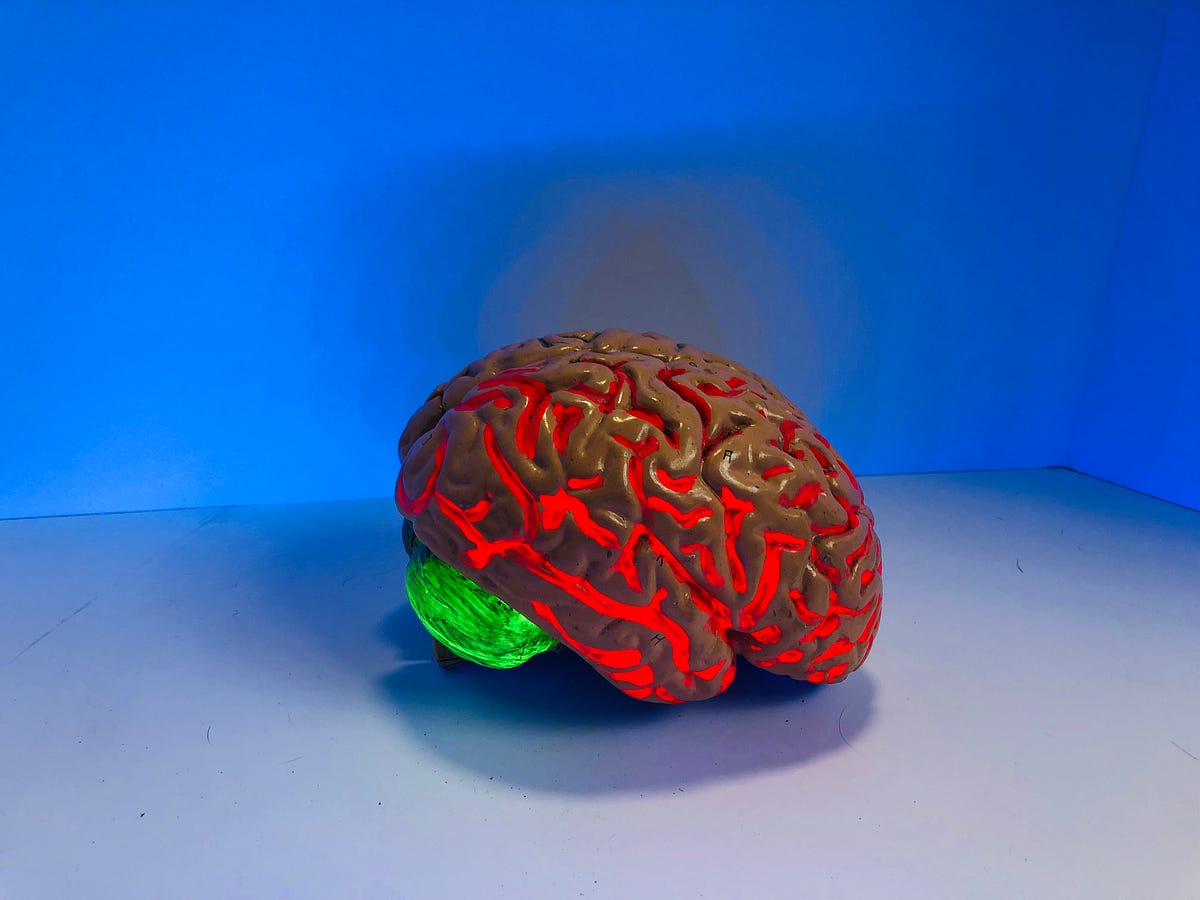# Build Your Own Neural Network From Scratch with PythonBuild Your Own Neural Network From Scratch with Python - There are many python libraries to build and train neural networks like Tensorflow and Keras. But to really understand neural networks, we need to understand its basic structure and be able to build and train a network of our own.

There are many python libraries to build and train neural networks like Tensorflow and Keras. But to really understand neural networks, we need to understand its basic structure and be able to build and train a network of our own. Neural networks can learn data much better compared to regular machine learning algorithms. In fact, a neural network algorithm can be interpreted as a bunch of linear regressions, where each node is an output of one linear regression...

## Deep Learning Tutorial with Python | Machine Learning with Neural Networks

In this video, Deep Learning Tutorial with Python | Machine Learning with Neural Networks Explained, Frank Kane helps de-mystify the world of deep learning and artificial neural networks with Python!

## Learn Machine Learning with Python (Part 3) | Machine Learning with Neural Networks

Learn Machine Learning with Python using neural networks with this machine learning beginners course. In this tutorial we will look at taking an existing sol...

## Deep Learning 101 —  Neural Networks Explained

The past few decades have witnessed a massive boom in the penetration as well as the power of computation, and amidst this information.

## Explain Deep Learning Neural Networks to your grandma

“You do not really understand something unless you can explain it to your grandmother” Not sure where this quote originally came from, it is sometimes kind of half-attributed to Albert Einstein.

## Deep Learning Explained in Layman's Terms

Deep Learning Explained in Layman's Terms. In this post, you will get to learn deep learning through a simple explanation (layman terms) and examples.